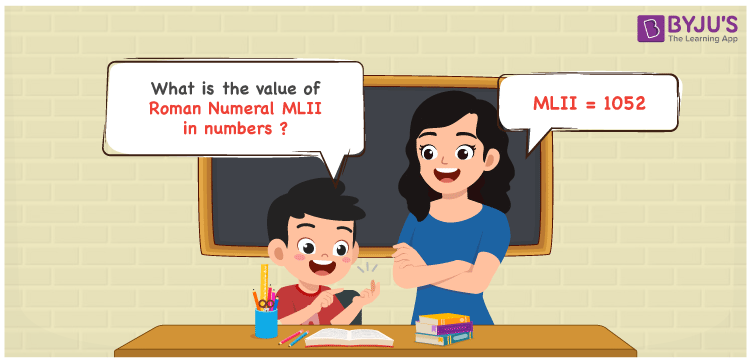Checkout JEE MAINS 2022 Question Paper Analysis : Checkout JEE MAINS 2022 Question Paper Analysis :

MLII Roman Numerals is 1052. The procedure followed to write MLII in numeric form is provided in a stepwise format in this article. The steps in the conversion of Roman numerals are explained in a simple to learn manner in order to increase the problem solving skills of primary school students. Hence, MLII is written in numeric form as 1052.

 Number Roman Numeral 1052 MLII## How to Write MLII Roman Numerals in Numbers?

Learn how MLII is expanded and shown in numbers using the steps available below for your guidance.

MLII = M + L + I + I

MLII = 1000 + 50 + 1 + 2

MLII = 1052

## Video Lesson on Roman Numerals## Frequently Asked Questions on MLII Roman Numerals

### Why can 1052 be written as MLII in Roman Numerals?

We know that;

M is the roman numeral of 1000

L is the roman numeral of 50

II is the roman numeral of 2

So MLII is the roman numeral of 1052.

### Calculate 1000 + 52.

We know that;

1000 + 52 = 1052

Hence, 1000 + 52 is 1052 which is written as MLII.

### What is (1000 – 1) + 53?

We know that

1000 – 1 = 999

999 + 53 = 1052

Therefore, (1000 – 1) + 53 is 1052.